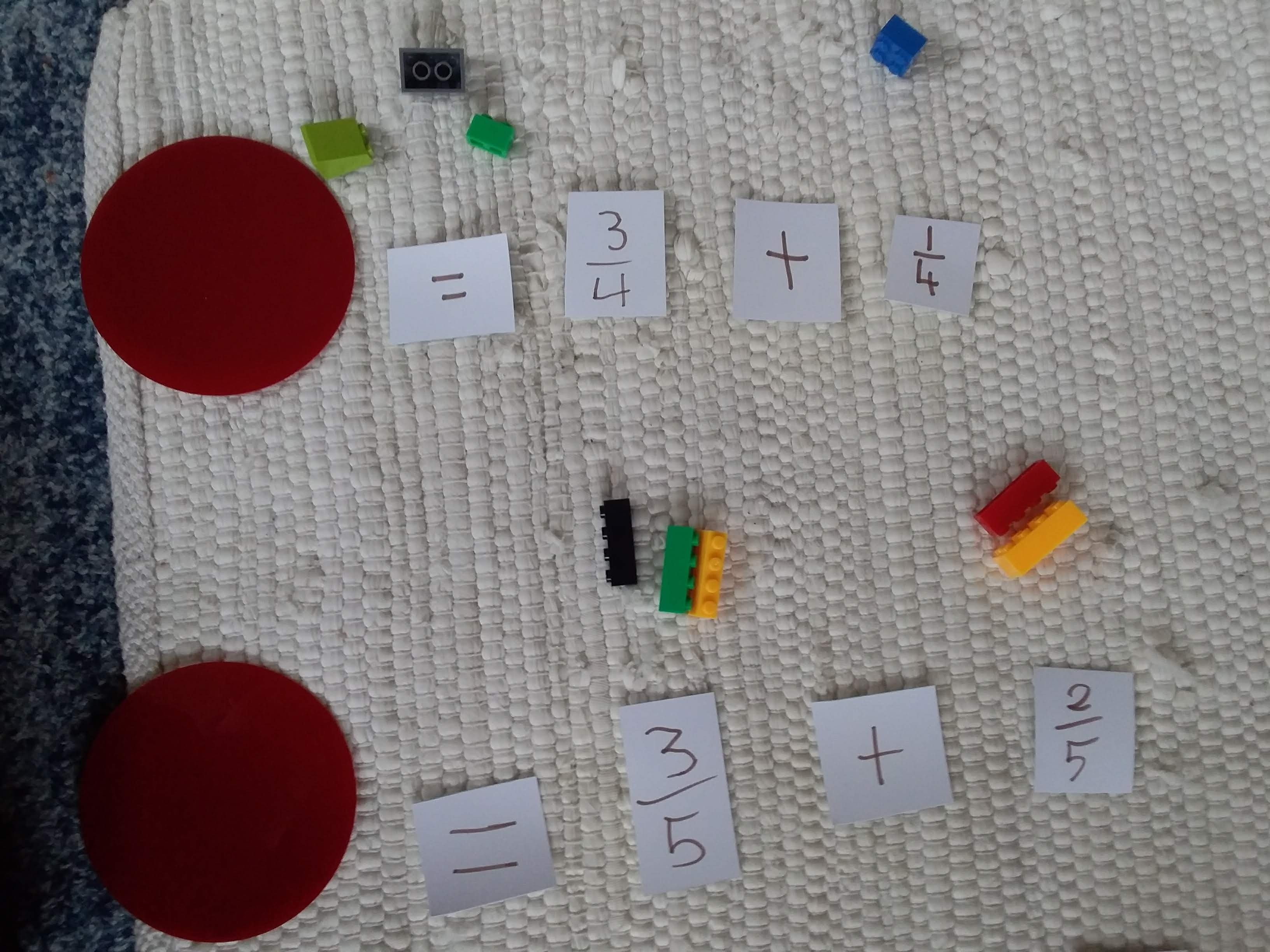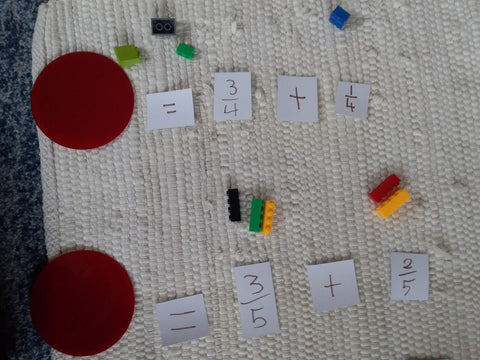# We need to talk about the equal sign

When you see an equal sign what do you think it means?

A lot of research of western educated children shows that many don't see it as meaning 'is the same as' but see it more as the thing that comes before the 'big' number in a sentence. I say western children as research from Ma (1999) cited in Darr (2001) says  “From the Chinese teachers’ perspective … the semantics of mathematical operations should be represented rigorously. It is intolerable to have two different values on each side of an equals sign. ”

Let's step back a minute and look at the history of the equal sign. Darr (2001) tells us it was first used to signify equality by Robert Recorde, an English mathematician. Recorde used two lines of the same length to mean "is the same as" because writing "is the same as" was 'tedious."

As I often tell my students Mathematicians look for short cuts. The down side of this is that many of us have internalised the short cut of the equal sign to mean "makes" rather than Recorde's initial use.

Matthews and Fuch (2018) carried out extensive research on children's knowledge of the equal sign. One of their findings was that a child's knowledge of the equal sign at second grade predicted their understanding of algebra when they were in fourth grade.  They found that second graders had more difficulty when an equation was written as _ = 3 + 2 rather than the often taught 3 + 2 = _

While the discussion here might seem trivial Matthews and Fuch say that understanding the "equality" aspect of the equal sign is a key for students being proficient in algebra and in their economic and academic future.

A recent report from the New Zealand Royal Society gave many recommendations on how maths teaching should be improved and looked at the social justice and economic issues involved in high quality maths teaching. One small aspect the report touched on was that teaching about the  equal sign needs to happen earlier in the curriculum (p.40)

So what are some ways to ensure that children understand the "is the same as" meaning of the equal sign?Darr says it is important we start early, as soon as children have been introduced to the symbols of maths.  Here are some of his other ideas:

- Work with students on another way of saying something on one side of an equation is the same as something on the other side, create a new symbol to mean 'is the same as."

-Present equations in different ways e.g. 4 + 5 = _ + 7

-True and False number sentences. Write a number sentence and have students say whether it is true or false and prove how they know the answer.

- Give an equation such as x + 2 = 5 and have the child say what they know about x. Is it going to be bigger or smaller than 5? How do they know?

-Write equations differently (as seen in the picture below) so that students know that the = sign isn't always at the end

- Talk about how the equal sign means balanced. You could balance objects on scales to show this.

If you are interested in a Montessori inspired activity to help with this check out this resource here

You may also be interested in:

Montessori Math Grid Game

Teaching Math Strategies in Montessori

## 1 comment

One of the few things my school did right in math. They presented us with a bunch of problems where the blank number could be anywhere in the equation.

Ettina